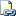# DATETIMEOFFSETFROMPARTS (Transact-SQL)

Returns a datetimeoffset value for the specified date and time and with the specified offsets and precision.Transact-SQL Syntax Conventions

## Syntax

``````DATETIMEOFFSETFROMPARTS ( year, month, day, hour, minute, seconds, fractions, hour_offset, minute_offset, precision )
``````

## Arguments

• year
Integer expression specifying a year.

• month
Integer expression specifying a month.

• day
Integer expression specifying a day.

• hour
Integer expression specifying hours.

• minute
Integer expression specifying minutes.

• seconds
Integer expression specifying seconds.

• fractions
Integer expression specifying fractions.

• hour_offset
Integer expression specifying the hour portion of the time zone offset.

• minute_offset
Integer expression specifying the minute portion of the time zone offset.

• precision
Integer literal specifying the precision of the datetimeoffset value to be returned.

## Return Types

datetimeoffset ( precision )

## Remarks

DATETIMEOFFSETFROMPARTS returns a fully initialized datetimeoffset data type. The offset arguments are used to represent the time zone offset. If the offset arguments are omitted, then the time zone offset is assumed to be 00:00, that is, there is no time zone offset. If the offset arguments are specified, then both arguments must be present and both must be positive or negative. If minute_offset is specified without hour_offset, an error is raised. If other arguments are not valid, then an error is raised. If required arguments are null, then a null is returned. However, if the precision argument is null, then an error is raised.

The fractions argument depends on the precision argument. For example, if precision is 7, then each fraction represents 100 nanoseconds; if precision is 3, then each fraction represents a millisecond. If the value of precision is zero, then the value of fractions must also be zero; otherwise, an error is raised.

This function is capable of being remoted to SQL Server 2012 servers and above. It will not be remoted to servers that have a version below SQL Server 2012.

## Examples

### A. Simple example without fractions of a second

``````SELECT DATETIMEOFFSETFROMPARTS ( 2010, 12, 31, 14, 23, 23, 0, 12, 0, 7 ) AS Result;
``````

Here is the result set.

``````Result
-------------------------------------------
2010-12-07 00:00:00.0000000 +00:00

(1 row(s) affected)
``````

### B. Example with fractions of a second

The following example demonstrates the use of the fractions and precision parameters:

1. When fractions has a value of 5 and precision has a value of 1, then the value of fractions represents 5/10 of a second.

2. When fractions has a value of 50 and precision has a value of 2, then the value of fractions represents 50/100 of a second.

3. When fractions has a value of 500 and precision has a value of 3, then the value of fractions represents 500/1000 of a second.

``````SELECT DATETIMEOFFSETFROMPARTS ( 2011, 8, 15, 14, 30, 00, 5, 12, 30, 1 );
SELECT DATETIMEOFFSETFROMPARTS ( 2011, 8, 15, 14, 30, 00, 50, 12, 30, 2 );
SELECT DATETIMEOFFSETFROMPARTS ( 2011, 8, 15, 14, 30, 00, 500, 12, 30, 3 );
GO
``````

Here is the result set.

``````----------------------------------
2011-08-15 14:30:00.5 +12:30

(1 row(s) affected)

----------------------------------
2011-08-15 14:30:00.50 +12:30

(1 row(s) affected)

----------------------------------
2011-08-15 14:30:00.500 +12:30

(1 row(s) affected)
``````